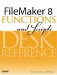# Round()

Category: Number

 Syntax: Round ( number; precision )

Parameters:

numberAny expression that resolves to a numeric value.

precisionAny number or numeric expression representing the number of decimal points to which to round the number.

Data type returned: Number

Description:

Returns the specified number rounded off to the number of decimal points specified by the precision parameter. The Round() function rounds numbers from 5 to 9 upward, and from 0 to 4 downward.

A precision of 0 rounds to the nearest integer. A negative number for the precision causes the number to be rounded to the nearest ten, hundred, thousand, and so on.

Examples:

Function

Results

Round (62.566; 2)

Returns 62.57.

Round (62.563; 2)

Returns 62.56.

Round (92.4; 0)

Returns 92.

Round (32343.98; -3)

Returns 32000.

Round (505.999; -1)

Returns 510.FileMaker 8 Functions and Scripts Desk Reference
ISBN: 0789735113
EAN: 2147483647
Year: 2004
Pages: 352